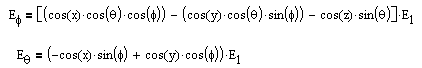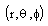# Source Code Description: Subroutine GFF

GFF

PURPOSE: to calculate the far-zone field of a sinusoidal electric monopole.

METHOD: If an electric line source has length d and endpoints at (x1, y1, z1) and (x2, y2, z2), then the coordinates of any point on the source are

x = x1 + l cos(x)

y = y1 + l cos(y)

z = z1 + l cos(z)

where cos(x), cos(y), cos(z) are the direction cosines of the l axis, and l is the distance along the source measured from the endpoint (x1, y1, z1). Let the current distribution on the monopole be:Where I1 and I2 are the endpoint currents. The far-zone field of this source is:whereandare the spherical coordinates of the observation point.

In this subroutine the range dependence has been suppressed. The far field vanishes in the endfire direction where GK=0. If a ground plane is present (IGRD > 0) the E1 equation above is decomposed into the x, y, and z components and the reflection coefficients are applied before Eq and Ef field components are returned to the calling program.

CALLED BY:

CALLS TO: NONE